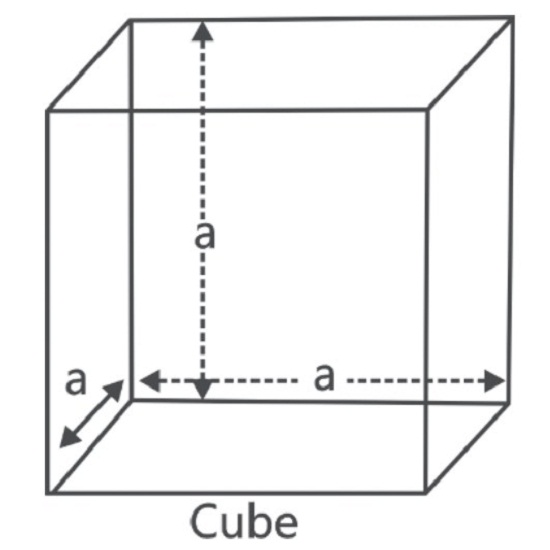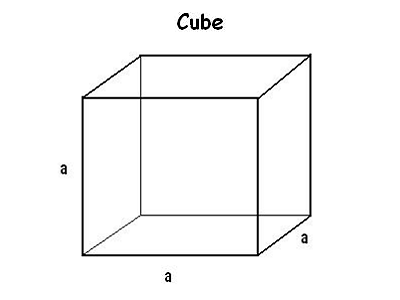# Volume of Cube Calculator

Cube surface area calculator is an online tool to calculate volume of cube given length of one side is known.

A cuboid whose length, breadth and height are all equal is called a cube. Cubes have 6 faces, 2 square bases, 4 square faces, 12 edges and 8 vertices. Examples of some common cube surface are: Ice-cubes, Sugar cubes, Dice, etc. are all examples of a cube. Each edge of a cube is called its side.

### Cube Formulae

Cube is a 3D object made up of 6 square faces, 8 vertices and 12 edges. All edges and faces are equal. It is also a prism because it has the same cross-section along a length. It is a square prism. All angles are 90°.For a cube of edge = a units, we have;

Sum of lengths of all edge = 12a units.

Diagonal of cube = (a ∛ 3) units

Total Surface Area of cube = (6a2) sq. units.

Lateral Surface Area of a cube = (4a2) sq. units

Volume of cube = a3 cubic units.

## Latest Blog topics in Maths### Spreading Knowledge Across the World

USA - United States of America  Canada  United Kingdom  Australia  New Zealand  South America  Brazil  Portugal  Netherland  South Africa  Ethiopia  Zambia  Singapore  Malaysia  India  China  UAE - Saudi Arabia  Qatar  Oman  Kuwait  Bahrain  Dubai  Israil  England  Scotland  Norway  Ireland  Denmark  France  Spain  Poland  and  many more....